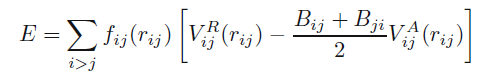# How to manipulate complex loss function?

Hi everyone,

I faced a problem to manipulate complex loss function. The following is my example. (A complex but simple one.)

I have N images and each image contains M atoms. Each atom has 13 features.

For each atom, after going through the neural network, it reports an atomic energy. I need to sum all of atoms in the same image up and compare with the ground truth (image energy).

For example:

I have 2 images with 5 and 9 atoms.

Input of NN = 14 x 13

Output of NN = 1 x 13

After summing up first 5 outputs and left 9 outputs. I will have 2 image energies.

These 2 images is “prediction” and will be used to calculate loss with ground truth “y”.

The codes are shown as follows:

========================================================================

class Net(torch.nn.Module):

``````def __init__(self, n_feature, n_hidden_1, n_hidden_2, n_output):
super(Net, self).__init__()
self.hidden_1 = torch.nn.Linear(n_feature , n_hidden_1)  # hidden layer
self.hidden_2 = torch.nn.Linear(n_hidden_1, n_hidden_2)  # hidden layer
self.predict  = torch.nn.Linear(n_hidden_2, n_output  )  # output layer

def forward(self, x):
x = torch.tanh(self.hidden_1(x))      # activation function for hidden layer
x = torch.tanh(self.hidden_2(x))      # activation function for hidden layer
x = self.predict(x)                   # linear output
return x
``````

x = Variable( torch.from_numpy( Descriptor_species ).float() )
y = Variable( torch.from_numpy( Data_energy).float() )

net = Net(n_feature=13, n_hidden_1=15, n_hidden_2=15, n_output=1) # define the network
loss_func = torch.nn.MSELoss() # this is for regression mean squared loss

for t in range(200):

``````prediction = []

for i in range(0,Number_total_images):
prediction += [sum(net(x)[Species_number_image[i] : Species_number_image[i+1]])]

loss = torch.sqrt(loss_func(prediction, y))     # must be (1. nn output, 2. target)
print(loss)

``````

========================================================================

The code reports an error message:

TypeError: expected Tensor as element 0 in argument 0, but got list

prediction = Variable ( torch.FloatTensor(prediction) , requires_grad = True )

before

loss = torch.sqrt(loss_func(prediction, y)) # must be (1. nn output, 2. target)

It works without any weight and bias updating.

Is any method to sum part of answer together and keep “requires_grad” as true?

========================================================================

The next goal is extending the output of NN as 8 neurons and set these 8 answers as 8 parameters. I will put these 8 parameters into the equation of bond order potential and calculate atomic energy for each atom. After that, the procedure will be as the same with previous problem.

The bond order potential equation looks like:Thanks for everyone’s help and suggestions.

I found a method but I think that it is not the best way to solve my question.

for t in range(2000):
prediction = net(x)

``````for i in range(0,Number_total_images):
prediction[i] = sum(net(x)[Species_number_image[i] : Species_number_image[i+1]])

loss = torch.sqrt(loss_func(prediction[0:Number_total_images], y))     # must be (1. nn output, 2. target)